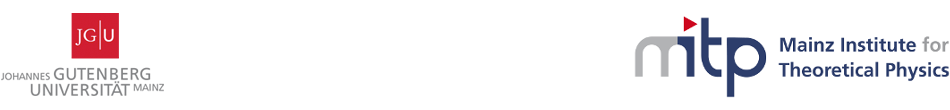#Geometry, Gravity and Supersymmetry

24-28 April 2017
Mainz Institute for Theoretical Physics, Johannes Gutenberg University
Europe/Berlin timezone

## Homogeneous solutions of decomposable 11D supergravity

27 Apr 2017, 11:05
45m
02.430 (Mainz Institute for Theoretical Physics, Johannes Gutenberg University)

### 02.430

#### Mainz Institute for Theoretical Physics, Johannes Gutenberg University

Staudingerweg 9 / 2nd floor, 55128 Mainz

### Speaker

Prof. Dmitri Alekseevsky (Moscow)

### Description

We will describe a class of solutions of 11 dimensional supergravity (M, g_M, F) under the assumption that the Lorentz manifold (M, g_M) is a direct product of a p-dimensional Lorentz manifold (M, g) and q = 11 − p - dimensional Riemann manifold (M ̄,g ̄). We made also some assumption about the structure of the 4-form F and write down and analyze the equa- tions of 11d supergravity in this case under assumption that the manifold (M,g_M,F) is homogeneous. In the case p = 4, we describe all such homo- geneous solutions of 11d decomposable supergravity under assumption that the 4-form is a direct sum of the volume form of a homogeneous Lorentz manifold (M,g) and an invariant 4-form of a compact homogeneous Riemannian manifold (M ̄ , g ̄). This is a joint work with I. Crysikos and A. Taghavi-Chabert.

### Primary author

Prof. Dmitri Alekseevsky (Moscow)

 Slides